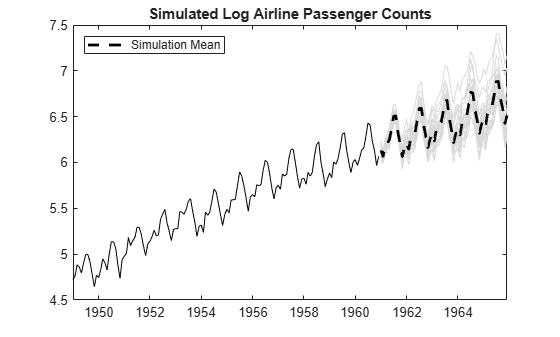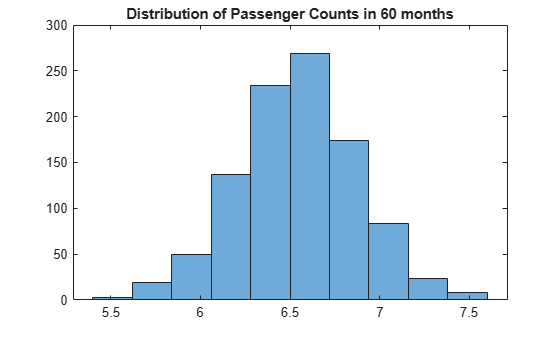# Simulate Multiplicative ARIMA Models

This example shows how to simulate sample paths from a multiplicative seasonal ARIMA model using `simulate`. The time series is monthly international airline passenger numbers from 1949 to 1960.

### Load Data and Estimate Model

Load the data set `Data_Airline`.

```load Data_Airline y = log(DataTimeTable.PSSG); T = length(y); Mdl = arima('Constant',0,'D',1,'Seasonality',12,... 'MALags',1,'SMALags',12); EstMdl = estimate(Mdl,y);```
``` ARIMA(0,1,1) Model Seasonally Integrated with Seasonal MA(12) (Gaussian Distribution): Value StandardError TStatistic PValue _________ _____________ __________ __________ Constant 0 0 NaN NaN MA{1} -0.37716 0.066794 -5.6466 1.6364e-08 SMA{12} -0.57238 0.085439 -6.6992 2.0952e-11 Variance 0.0012634 0.00012395 10.193 2.1406e-24 ```
`res = infer(EstMdl,y);`

### Simulate Airline Passenger Counts

Use the fitted model to simulate 25 realizations of airline passenger counts over a 60-month (5-year) horizon. Use the observed series and inferred residuals as presample data.

```rng('default') Ysim = simulate(EstMdl,60,'NumPaths',25,'Y0',y,'E0',res); mn = mean(Ysim,2); fh = DataTimeTable.Time(end) + calmonths(1:60); figure plot(DataTimeTable.Time,y,'k') hold on plot(fh,Ysim,'Color',[.85,.85,.85]); h = plot(fh,mn,'k--','LineWidth',2); title('Simulated Airline Passenger Counts') legend(h,'Simulation Mean','Location','NorthWest') hold off```The simulated forecasts show growth and seasonal periodicity similar to the observed series.

### Estimate Probability of Future Event

Use simulations to estimate the probability that log airline passenger counts will meet or exceed the value 7 sometime during the next 5 years. Calculate the Monte Carlo error associated with the estimated probability.

```Ysim = simulate(EstMdl,60,'NumPaths',1000,'Y0',y,'E0',res); g7 = sum(Ysim >= 7) > 0; phat = mean(g7)```
```phat = 0.3820 ```
`err = sqrt(phat*(1-phat)/1000)`
```err = 0.0154 ```

The probability that the (log) number of airline passengers will meet or exceed 7 in the next 5 years is approximately a 0.38. The Monte Carlo standard error of the estimate is about 0.02.

### Plot the Distribution of Passengers at a Future Time.

Use the simulations to plot the distribution of (log) airline passenger counts 60 months into the future.

```figure histogram(Ysim(60,:),10) title('Distribution of Passenger Counts in 60 months')```